# Circuit Analysis

## Part 1

You work in the quality control department of a microchip factory and your job is to help identify which machines are creating erroneous circuit boards so that those machines can be fixed or replaced. The circuit boards your company produces are made up of three components: the board itself, pins, and wires which connect pins to each other. To help you identify erroneous circuits, you are given a reference sheet for each board that the factory prints which tells you which pins must be connected to ensure that it will function properly.

In this assignment, your input is:

• A picture of a circuit board, where blue pixels represent pins and black pixels represent wires. White pixels are simply points where the board does not have either a pin or a wire. Both pins and wires are conductive, meaning that electricity can flow through them, but the board itself is not.

• A list of connections which must exist in order for the board to function properly, where each connection is represented by a struct containing two pins. Pins are identified by `Posn\$` structs corresponding to their position on the circuit board. For example, `(make-posn 0 0)` identifies the pin in the upper left corner of the image. There may be pins on the board which are not included in any connection in the connections list.

Two points on the circuit board are considered connected if electricity can flow between the points. This is true only if both of the following conditions are met:

• The two points are both conductive.

• There is a chain of orthogonally adjacent conductive pixels between them in the picture.

Please note that diagonally adjacent conductive pixels are not necessarily connected. Also note that connections are transitive. In other words, if connections `A-B` and `B-C` exist, then connection `A-C` is implicitly present as well. Therefore, there may be connections which are necessary for the circuit to function that only appear implicitly on the reference sheet. However, connections which are neither implicitly nor explicitly specified by the reference sheet will cause an error.

Your program should output all errors which exist in the circuit board. Faults occur whenever pins in the circuit are connected when they should not be or are not connected when they should be. Although it would be simple to output a list of pairs which do not match the specification, this would result in a great deal of redundancy. Consider the case where pins `A, B, C, D, E,` and `F` are all supposed to be mutually connected. The circuit

`A-B-C-D-E-F`

clearly satisfies this. However, if we take away the middle connection, the circuit

`A-B-C D-E-F`

now has nine pairs of pins (`A-D, A-E, A-F, B-D,` etc.) that are not connected with each other, even though only one wire was removed. This should really only be one fault, not nine.

At this point, it will be useful to notice that the set of pins on a board can be separated into groups based on their connections. For instance, in the board above, the specification sheet said that the pins should all be in one group `(A,B,C,D,E,F)`, whereas the board had them split into groups `(A,B,C)` and `(D,E,F)`. These groups of mutually connected pins will be referred to as islands.

We will describe each type of fault in terms of islands in the circuit we are verifying:

• An open circuit occurs when two separate islands in the circuit contain pins that should be connected according to the reference sheet. Note that 'circuit' refers to the actual circuit represented by the image.

• A short circuit occurs when an island in the circuit contains pins that should not be connected according to the reference sheet.

To clarify, here is a brief example displaying both types of faults. This picture shows how the pins should be grouped based on their reference sheet (the boxes show islands):and this picture shows how they are actually connected in the manufactured circuit: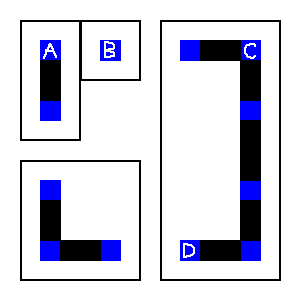In this example, there is an open circuit between the island containing pin `A` and the island containing pin `B` because `A` and `B` should be be connected, and there is a short circuit in the island containing `C` because pins `C` and `D` should not be connected. There are no other faults in this example.

Your program must return a complete list of faults containing every open and short circuit exactly once. You can use any pin contained in an island to represent it (we arbitrarily chose `A, B,` and `C` above). You should build your list of faults using the following constructors:

• `(make-open-circuit pin1 pin2)` creates a fault for an open circuit between the islands containing the given pins. Note that `pin1` and `pin2` do not necessarily need to be the pins that should be connected, so long as the pins that should be connected are in the same islands as `pin1` and `pin2`, respectively.

• `(make-short-circuit pin)` creates a fault for a short circuit within the island containing the given pin.

The order of the list of faults does not matter, but it must contain exactly only one element for each fault.

The following examples illustrate some possible inputs and outputs. Note that other outputs are possible, since there are several possible representative choices for each island. The circuits are enlarged versions of the sorts of images your program should accept as input (see support code below), and the output is in the format we expect. It would be worthwhile to ensure your solution outputs similar results for all of the following examples, since if it doesn't you are not adhering to the spec and will lose points.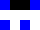Connection list:

`(list (make-connection (make-posn 0 2) (make-posn 2 2)))`

Output:

```(list (make-open-circuit (make-posn 0 2) (make-posn 2 2))
(make-short-circuit (make-posn 0 0)))
```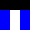Connection list:

```(list (make-connection (make-posn 0 1) (make-posn 2 1))
(make-connection (make-posn 2 1) (make-posn 0 2))
(make-connection (make-posn 0 2) (make-posn 2 2)))
```

Output:
`empty`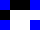Connection list:

```(list (make-connection (make-posn 0 0) (make-posn 3 2))
(make-connection (make-posn 0 2) (make-posn 3 0)))
```

Output:

```(list (make-open-circuit (make-posn 0 2) (make-posn 3 2))
(make-short-circuit (make-posn 0 2)))
```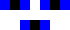Connection list:

```(list (make-connection (make-posn 0 0) (make-posn 4 0))
(make-connection (make-posn 4 0) (make-posn 2 2))
(make-connection (make-posn 6 0) (make-posn 4 2))
(make-connection (make-posn 2 0) (make-posn 4 2)))
```

Output:

```(list (make-short-circuit (make-posn 2 0))
(make-short-circuit (make-posn 2 2))
(make-short-circuit (make-posn 4 0))
(make-open-circuit (make-posn 2 0) (make-posn 4 0))
(make-open-circuit (make-posn 6 0) (make-posn 2 2))
(make-open-circuit (make-posn 2 0) (make-posn 4 2)))
```Connection list:

```(list (make-connection (make-posn 0 0) (make-posn 1 2))
(make-connection (make-posn 2 0) (make-posn 3 2))
(make-connection (make-posn 4 0) (make-posn 1 2))
(make-connection (make-posn 0 0) (make-posn 2 0)))
```

Output:

```(list (make-open-circuit (make-posn 0 0) (make-posn 2 0))
(make-open-circuit (make-posn 0 0) (make-posn 4 0))
(make-open-circuit (make-posn 0 0) (make-posn 1 2))
(make-open-circuit (make-posn 0 0) (make-posn 3 2))
(make-open-circuit (make-posn 2 0) (make-posn 4 0))
(make-open-circuit (make-posn 2 0) (make-posn 1 2))
(make-open-circuit (make-posn 2 0) (make-posn 3 2))
(make-open-circuit (make-posn 4 0) (make-posn 1 2))
(make-open-circuit (make-posn 4 0) (make-posn 3 2))
(make-open-circuit (make-posn 1 2) (make-posn 3 2)))
```

We have provided some support code in the circuit-support.rkt file. If you download and then require it, it will help you manage the circuit images. The following structs and functions are defined in the support code:

```(define-struct posn (x y))
(define-struct circuit-node (color))
(define-struct connection (pin1 pin2))
(define-struct short-circuit (pin))
(define-struct open-circuit (pin1 pin2))
```

`circuit-node` is a struct used by the helper fuctions below. Its `color` is either `'black`, `'white`, or `'blue`.

Note that while the contracts below follow the contracts built into the `cs019` lang, the signatures (`Posn\$`, `CircuitNode\$`, etc) are not defined by the support code. It is your responsibility to define these.

• `ternary->circuit : ((Listof: (Listof: Integer\$)) -> (Listof: (Listof: CircuitNode\$)))`
Converts a ternary representation of a circuit into a circuit-node represenation by changing `2` to `'blue`, `1` to `'black`, and `0` to `'white`. This function should be helpful when creating your own test circuits.

• `image->circuit : (Image\$ -> (Listof: (Listof: CircuitNode\$)))`
Converts a circuit board image to a list of lists of circuit-nodes.

• `bigimage->circuit : (Image\$ -> (Listof: (Listof: CircuitNode\$)))`
Converts a magnified circuit board image (where every 10x10px square represents a single pixel) to a list of list of circuit-nodes.

• `circuit->image : ((Listof: (Listof: CircuitNode\$)) -> Image\$)`
Takes a circuit and generates a circuit board image.

• `circuit->bigimage : ((Listof: (Listof: CircuitNode\$)) -> Image\$)`
Takes a circuit and generates a magnified circuit board image. This function is very helpful if you want to be able to see the circuit board images you are working with.

• `random-circuit : (Integer\$ Integer\$ Number\$ Number\$ -> (Listof: (Listof: CircuitNode\$)))`
Generates a random circuit with height and width specified by the first two arguments. The third argument specifies the probability (between 0 and 1) that each pixel is conducting, and the fourth specifies the probability (between 0 and 1) that each conducting pixel contains a pin.

The entry point to your program should have the following signature:

```analyze-circuit-image : (Image\$ (Listof: Connection\$) -> (Listof: (or: OpenCircuit\$ ShortCircuit\$))```

Here are a few test circuit board images:

• How do you find islands?
• How do you detect open circuits?
• How do you detect short circuits?

## Part 2

Run your solution to part one on `big_circuit.png` and the list of connections defined as `big-connections` in the support code.

You have probably noticed that it is taking a while. Think about why this is.

Run your program on `big_circuit.png` again, but with a much smaller list of connections. Now remove many of the pins in `big_circuit.png` (using an image editor or `circuit->image`). Run your program again (with a correspondingly smaller connections list). Think about how these changes affect the overall runtime of the program.

Give a big-O analysis of your program. Be sure to talk about each of the different parts of your code separately, and to clearly define any variables you use in your analysis.

## Part 3

Do you think this problem can be solved more efficiently? Identify the bottlenecks in the strategy you use, and discuss possible strategies for improving them.

## What to turn in:

• A Racket file, `circuit-analysis.rkt`, containing your full solution to part 1.

• A file, `readme.[txt,pdf,doc]`, containing answers to the questions in part 1.

• A file, `analysis.[txt,pdf,doc`] with your answers for Parts 2 and 3.
As always, these files must be in plain text, pdf, or, if you really must, Word format. If you use Word, we will accept only Word 2003 (`.doc`) files. If you are using Word 2007, you should export to a pdf. We will not accept Word 2007 (`.docx`) files because we cannot read them.